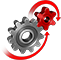# WolframSystemModeler

## Cam Design: Create Automotive Components in the Wolfram Language

Cams are used in a number of different mechanisms, from valve actuation in internal combustion engines to sewing machines. With the symbolic component creation capabilities in SystemModeler, it's easy to design and prototype mechanisms with correct displacements and minimal wear.

To run this example, you'll need

Prototype equations in the Wolfram Language

Mathematica is used to define the shape of three different cams, one pointy, one with a full rise harmonic shape, and one in between.

Derive differential equations with the Wolfram Language

Describe the physical behavior of the system with symbolic differential equations.Connect Components

Create structural models from Mathematica, enabling rapid prototyping of different system configurations.

Visualize the movement

Directly combine simulation results with graphic constructs in the Wolfram Language to communicate model characteristics.

Watch the VideoComponent created based on the equations.
Cam driven by a torque and connected to a follower.
Cam designers want to minimize the jerk (the derivative of the acceleration), in order to minimize the stress and wear on the cam.
Animation of the cam followers created in Mathematica.

Create components based on Wolfram Language expressions

Create reusable Modelica components based on equations in the Wolfram Language for direct use in SystemModeler.

Analyze the jerk for different cam options

Directly analyze and visualize simulation results in Mathematica.

## Wolfram System Modeler

Questions? Comments? Contact a Wolfram expert »